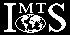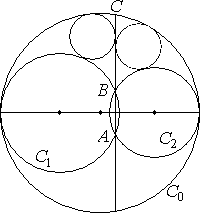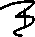# International Mathematical Talent Search

IMTS Rounds Index: 1ñ9 10ñ19 20

##Problems Round 20

### Problem 1/20

Determine the number of points (x, y) on the hyperbola

2xy ñ 5x + y = 55

for which both x and y are integers.

### Problem 2/20

Find the smallest value of n for which the following statement is true: Out of every set of n positive integers one can always choose seven numbers whose sum is divisible by 7.

### Problem 3/20

The husbands of 11 mathematicians accompany their wives to a meeting. Sometimes the husbands pass one another in the halls, but once any particular pair have passed each other once, they never pass each other again. When they pass one another, either only one of them recognizes the other, or they mutually recognize each other, or neither recognizes the other. We will refer to the event of one husband recognizing another one as a ìsightingî, and to the event of them mutually recognizing each other as a ìchatî, since in that case they stop for a chat. Note that each chat accounts for two sightings.

If 61 sightings take place, prove that one of the husbands must have had at least two chats.

### Problem 4/20

Suppose that a and b are positive integers such that the fractions a / (b ñ 1) and a / b, when rounded (by the usual rule; i. e., digits 5 and larger are rounded up, while digits 4 and smaller are rounded down) to three decimal places, both have the decimal value 0.333.

Find, with proof, the smallest possible value of b.

### Problem 5/20In the figure shown on the right, the centers of circles C0, C1, and C2 are collinear, A and B are the points of intersection of C1 and C2, and C is point of intersection of C0 and the extension of AB. Prove that the two small circles shown, tangent to C0, C1 and BC, and C0, C2 and BC, respectively, are congruent to one another.

IMTS Rounds Index: 1ñ9 10ñ19 20
Prepared by Vladimirensa. Last updated on 2nd August, 1998.